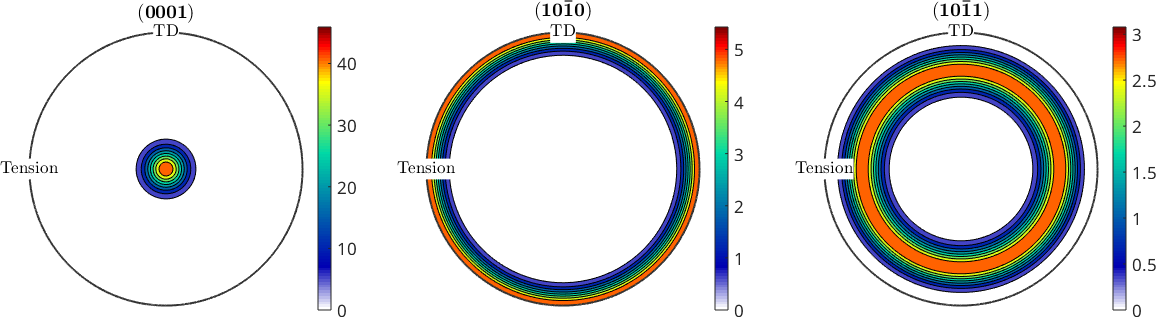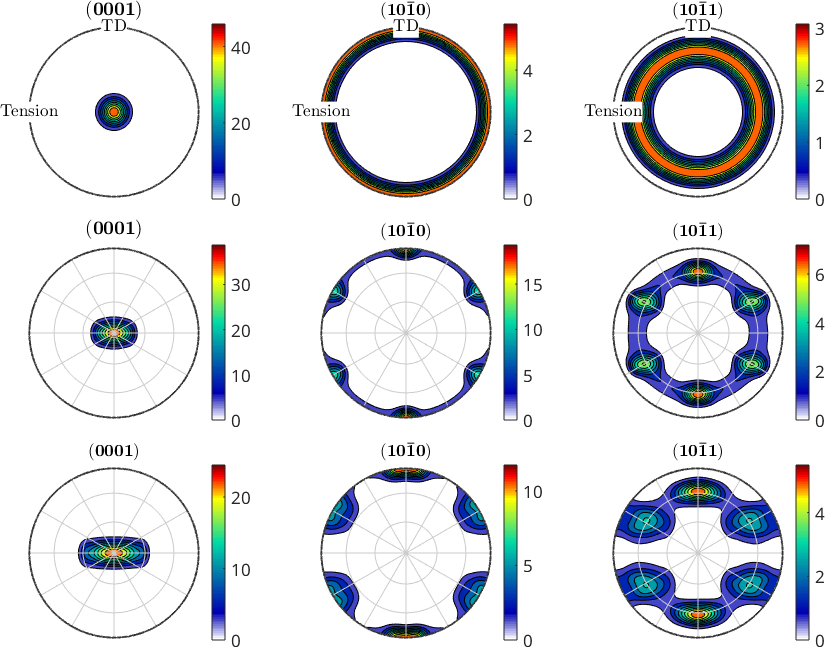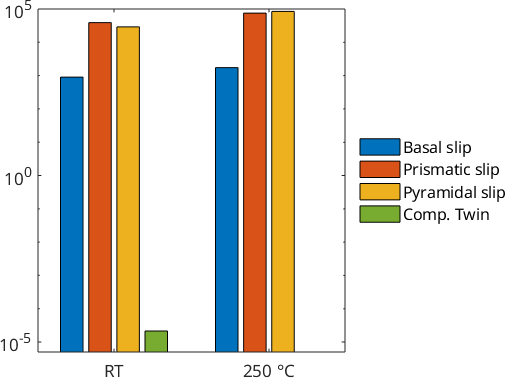Texture evolution in rolled magnesium during uniaxial tension edit page

This examples models the texture evolution of rolled magnesium under unixaxial tension using the Taylor model. The undeformed material is assumed to have a basal fibre texture perpendicular to tension direction. Then tension experiment has been performed twice: at room temperature and at 250 degree Celcius. The strain at fracture was approx. 30 percent and 70 percent, respectively.

## Setting up hexagonal crystal symmetry

First we need to set up hexagonal crystal symmetry.

## Setting up the basal fibre texture

Second, we set up the initial fibre texture which has the c-axis perpendicular to the (x,y)-sheet plane and the a-axes are randomized. This is typical for rolled Mg-sheet

## Plot polefigures of generated initial state without strains

define crystal orientations of interest for polefigures and plot figure## Setting up the slip systems

The critical resolved shear stresses (CRSS) needed to activate certain slip systems is temperature AND material dependant. As it is not trivial to measure/define CRSS, there are many different values in literature.

In practise, at room temperature basal slip dominates and commonly simple tension twins are activated as well (they have very low CRSS). During tension stress perpendicular to the c-axis tension twinning cannot occur. Thats why it is sensible to only define compression twins (which in Mg have a very high CRSS)

At higher temperatures the CRSS of non-basal slip systems decreases.

## Defining strain tensors

Due to constant volume law, the sum of all strains must equal zero. Here slightly anisotropic strain is assumed at room temperature, with more thinning in y-direction than in z-direction. In practise the anisotropy of Mg at high temperatures becomes negligible.

## Calculate texture evolution

The Tayor calculation is used to get the resulting spin of each vector as well as the coeffeicients for each slip system for cold and hot state

Apply the Taylor spin to the initial orientation distribution

Plot pole figures in comparison to inital texture## Statistics on activated slip systems

By adding up the coefficients of the taylor calculation and grouping them according to their slip system type, a bar chart can be plotted

The results can be plotted with logarithmic scale for better visualization